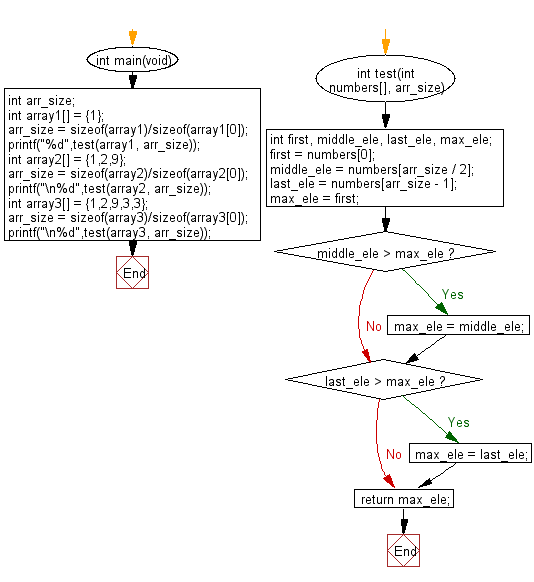﻿ C : First, last, and middle of an array with odd lengths# C Exercises: Find the largest value from first, last, and middle elements of a given array of integers of odd length

## C-programming basic algorithm: Exercise-50 with Solution

Write a C program to find the largest value from first, last, and middle elements of a given array of integers of odd length (atleast 1).

C Code:

``````#include <stdio.h>
#include <stdlib.h>

int main(void){
int arr_size;
int array1[] = {1};
arr_size = sizeof(array1)/sizeof(array1);
printf("%d",test(array1, arr_size));
int array2[] = {1,2,9};
arr_size = sizeof(array2)/sizeof(array2);
printf("\n%d",test(array2, arr_size));
int array3[] = {1,2,9,3,3};
arr_size = sizeof(array3)/sizeof(array3);
printf("\n%d",test(array3, arr_size));
}
int test(int numbers[], arr_size)
{
int first, middle_ele, last_ele, max_ele;
first = numbers;
middle_ele = numbers[arr_size / 2];
last_ele = numbers[arr_size - 1];
max_ele = first;

if (middle_ele > max_ele)
{
max_ele = middle_ele;
}
if (last_ele > max_ele)
{
max_ele = last_ele;
}
return max_ele;
}
``````

Sample Output:

```1
9
9
```

Pictorial Presentation:Flowchart:C Programming Code Editor:

What is the difficulty level of this exercise?

Test your Programming skills with w3resource's quiz.

﻿

## C Programming: Tips of the Day

Why doesn't a+++++b work?

printf("%d",a+++++b); is interpreted as (a++)++ + b according to the Maximal Munch Rule!.

++ (postfix) doesn't evaluate to an lvalue but it requires its operand to be an lvalue.

! 6.4/4 says the next preprocessing token is the longest sequence of characters that could constitute a preprocessing token"

Ref : https://bit.ly/3fdldUT